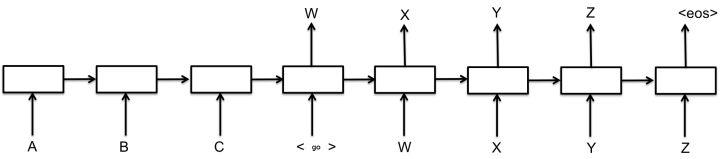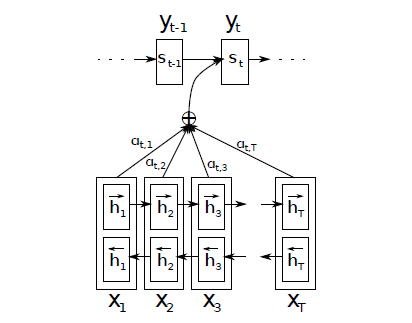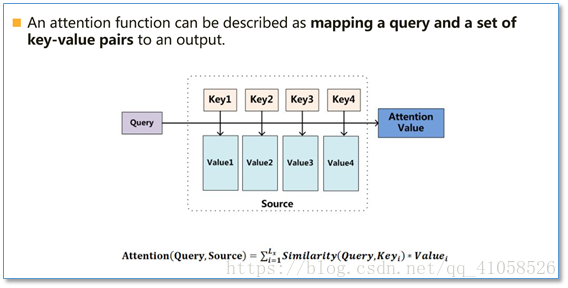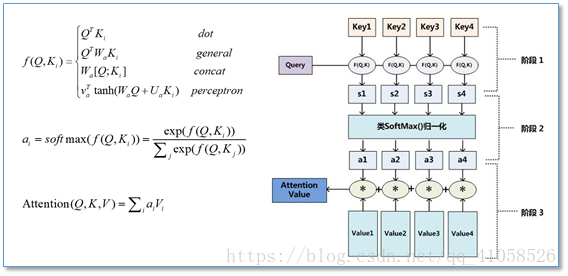# Seq2Seq模型

## 基本原理

• 核心思想：将一个作为输入的序列映射为一个作为输出的序列

• 编码输入
• 解码输出
•• 解码第一步，解码器进入编码器的最终状态，生成第一个输出
• 以后解码器读入上一步的输出，生成当前步输出
• 组成部件：

• Encoder
• Decoder
• 连接两者的固定大小的State Vector

## 解码方法

• 最核心部分，大部分的改进
• 贪心法
• 选取一种度量标准后，在当前状态下选择最佳的一个结果，直到结束
• 计算代价低
• 局部最优解
• 集束搜索(Beam Search)
• 启发式算法
• 保存beam size个当前较佳选择,决定了计算量，8~12最佳
• 解码时每一步根据保存的结果选择下一步扩展和排序，选择前beam size个保存
• 循环迭代，直到结束。选择最佳结果输出
• 改进
• 堆叠RNN
• Dropout机制
• 与编码器之间建立残差连接
• 注意力机制
• 记忆网络

# 注意力机制

## Seq2Seq模型中的注意力机制

• 在实际发现，随着输入序列增长，模型性能发生显著下降
• 小技巧
• 将源语言句子逆序输入，或者重复输入两遍，得到一定的性能提升
• 解码时当前词及对应的源语言词的上下文信息和位置信息在编解码过程中丢失了
• 引入注意力机制解决上述问题：
• 解码时，每一个输出词都依赖于前一个隐状态以及输入序列每一个对应的隐状态

$s_i = f(s_{i-1}, y_{i-1},c_i)$

$p(y_i|y_1,\cdots,y_{i-1})=g(y_{i-1},s_i,c_i)$

其中，$y$是输出词，$s$是当前隐状态，$f,g$是非线性变换，通常为神经网络
• 语境向量$c_i$是输入序列全部隐状态$h_1,\cdots,h_T$的加权和

$c_i=\sum \limits_{j=1}^T a_{ij}h_j$

$a_{ij} = \frac{\exp(e_{ij})}{\sum_k \exp(e_{ij})}$

$e_{ij}=a(s_{i-1},h_j)$

• 神经网络$a$将上一个输出序列隐状态$s_{i-1}$和输入序列隐状态$h_j$作为输入，计算出一个$x_j,y_i$对齐的值$e_{ij}$
• 考虑每个输入词与当前输出词的对齐关系，对齐越好的词，会有更大权重，对当前输出影响更大
• 双向循环神经网络
• 单方向：$h_i$只包含了$x_0$$x_i$的信息，$a_{ij}$丢失了$x_i$后面的信息

• 双方向：第$i$个输入词对应的隐状态包括了$\overrightarrow{h}_i$$\overleftarrow{h}_i$，前者编码了$x_0$$x_i$的信息，后者编码了$x_i$及之后的信息，防止信息丢失## 常见Attention形式

• 本质：一个查询（query）到一系列（键key-值value）对的映射• 计算过程

• 将query和每个key进行相似度计算得到权重，常用的相似度函数有点积，拼接，感知机等
• 使用一个softmax函数对这些权重进行归一化
• 权重和相应的键值value进行加权求和得到最后的attentionposted @ 2019-11-24 16:40  WeilongHu  阅读(1247)  评论(0编辑  收藏  举报